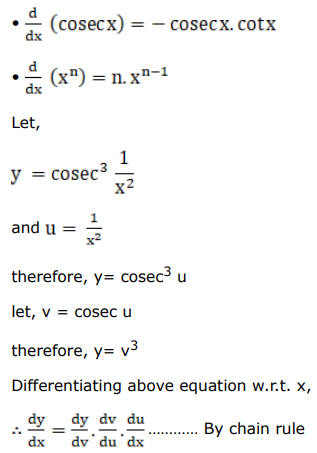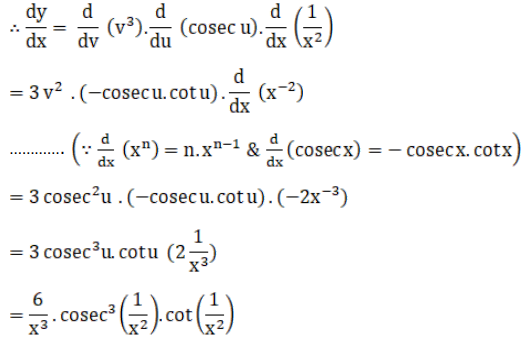# Differentiate each of the following w.r.t.Question:

Differentiate each of the following w.r.t. $x$ :

$\operatorname{cosec}^{3} \frac{1}{x^{2}}$

Solution: